# At a certain temperature. the equilibrium constant. kc. for this reaction is 53.3.

H2 + I2 —> 2HI
<--- at this temperature, 0.800 mol of H2 AND .800 mol of I2 were placed in 1.00L container to react. What concentration of HI is present at equilibrium? [HI]= ?M I'm stuck on what to do after the ICE chart.

General guidance

Concepts and reason

The concepts used to solve this problem chemical equilibrium.
A chemical reaction attains the stage of equilibrium when carried out in a closed vessel. In chemical equilibrium, the rate of the forward reaction becomes equal to the rate of backward reaction which results in no net changes in the concentration of reactants and products.

Fundamentals

As defined by the Law of chemical equilibrium, the ratio of the product of the reaction product raised to their respective stoichiometric coefficient to the product of the concentration of reactant raised to the respective stoichiometric coefficient has a constant value. The constant value is known as equilibrium constant. For the reaction, equilibrium constant is denoted by .
Consider the following equation for a gas-phase reaction:

Using Law of chemical equilibrium, the relation between concentration of reactant and product is:

Concentration of compounds in solution can be calculated by using the relation as:

Here, c is the concentration, n is the moles and V is the volume of the reaction vessel.

Step-by-step

Step 1 of 2

Consider the reaction:

Initial concentration of is and is . is 53.3
ICE table for the reaction is:

Use the expression:

Substitute 53.3 for , for and 0.80 for in the above equation for the calculation of as:

On solving, the equation is:

The value of x is solved by using the quadratic equation:

Substitute -85.28 for b, 49.3 for a and 34.112 for c and solve the equation:

The values for x is 0.63 or 1.10.

If be the decrease in concentration of and at time t and two mole each of is being formed, the increase in concentration of is . The value of x cannot be more than the initial concentration of reactants. Therefore, the value of is 0.63

Calculate the concentration of product by referring ICE table.

Also Read :   does getting punched in the jejunum really make you throw up?

Step 2 of 2

As per the ICE table:
Calculate number of moles of at equilibrium by using the relation as:

Substitute 0.63 for x in the above equation for the calculation of as:

Calculate in by using the relation as:

Substitute
Therefore, for V and for n in the above equation for the calculation of c as:

Therefore, the concentration of is

The concentration of is

According to the ICE table, is present as . The value of x is 0.63. By substituting the value of x, moles of are calculated. Concentration of is calculated by using the relation as:

Therefore, is .

Do not consider x as . Also, do not use the relation for the calculation of moles of . The correct unit of concentration is .

The concentration of is

The concentration of is

k
A2 +B2 2AB
[AB] k A,J[B,J
п C
Н, +1, 2HI
2
0.800 mol
2
0.800 mol
Concentration(mol) 2HI Н, Initial 0.800 0.800 Change +2x -x x 0.800 x 0.800-x Equilibrium 2x
HIP k H2JI,] X
0.80
2
2
2x]j° 53.3 (0.800-x]x[0.800-x] X 53.3(0.800-x) 4x 53.3(0.64+x+1.6x) 4x 34.112+53.3×2-85.28x=4×2
49.3×2-85.28x+34.112 = 0
-btb2-4ac X = 2а
-(-85.28)t (85.28)*-4x(49.3)x(34.112) X = – 2×49.3
х mol хт
2
2
HI
HI
2x
HI
[HI 2x X
Пи
П 2×0.63 =1.26
HI
1.00 L
п C
1.00 L
1.26 mol
1.26 mol n C 1.00 L =1.26 mol L
HI
1.26 mol L
HI
2x
HI
HI
п C
HI
1.26 mol L
1.10
0.800+2x
HI
mol L
HI
1.26 mol L
HI
1.26 mol L
We were unable to transcribe this image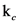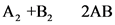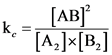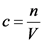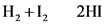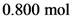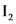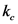2HI
<--- at this temperature, 0.800 mol of H2 AND .800 mol of I2 were placed in 1.00L container to react. What concentration of HI is present at equilibrium? [HI]= ?M Im stuck on what to do after the ICE chart.'/>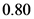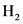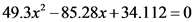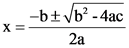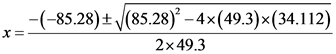2HI
<--- at this temperature, 0.800 mol of H2 AND .800 mol of I2 were placed in 1.00L container to react. What concentration of HI is present at equilibrium? [HI]= ?M Im stuck on what to do after the ICE chart.'/>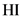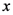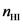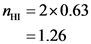2HI
<--- at this temperature, 0.800 mol of H2 AND .800 mol of I2 were placed in 1.00L container to react. What concentration of HI is present at equilibrium? [HI]= ?M Im stuck on what to do after the ICE chart.'/>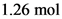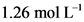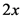2HI
<--- at this temperature, 0.800 mol of H2 AND .800 mol of I2 were placed in 1.00L container to react. What concentration of HI is present at equilibrium? [HI]= ?M Im stuck on what to do after the ICE chart.'/>2HI
<--- at this temperature, 0.800 mol of H2 AND .800 mol of I2 were placed in 1.00L container to react. What concentration of HI is present at equilibrium? [HI]= ?M Im stuck on what to do after the ICE chart.'/>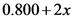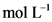Also Read :   Fast egg hatch action replay code for pokemon heart gold?